# Exponentiation - math problems

If n is a positive integer and x is any real number, then x^n corresponds to repeated multiplication x^n=x×x×⋯×x (n times). We can call this “x raised to the power of n,” “x to the power of n,” or simply “x to the n.” Here, x is the base, and n is the exponent or the power.

#### Number of problems found: 126

• Future value investmentPoseidon deposited 2,747 golden drachmas in a Mount Olympus college savings account to ensure Percy can go to college. It pays 0.04 (percent in decimal form) annual interest. After 11 years, he withdraws the money. How much more money would he have if the
• The town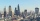The town population is 56000. It is decreasing by 2% every year. What will be the population of the town after 13 years?
• Maturity valueIKEA Furniture’s can purchase furniture with 3 year simple interest loan at 8% interest per year. What is the maturity value of 3500 loan?
• Two years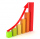Roy deposited 50,000.00 into his account paying 4% annual interest compounded semi annually. How much is the interest after 2 years?
• Annual increaseThe number of cars produced increased from 45,000 to 47,000 in 3 years. Calculate the average annual increase in cars in%.
• If you 3If you deposit \$4500 at 5% annual interest compound quarterly, how much money will be in the account after 10 years?
• What is 10What is the 5th term, if the 8th term is 80 and common ratio r =1/2?
• If the 3If the 6th term of a GP is 4 and the 10th is 4/81, find common ratio r.
• Percentage increaseWhat is the annual percentage increase in the city when the population has tripled in 20 years?
• All use computerIt is reported that 72% of working women use computers at work. Choose 3 women at random, find the probability that all 3 women use a computer in their jobs.
• The half lifeThe half-life of a radioactive isotope is the time it takes for a quantity of the isotope to be reduced to half its initial mass. Starting with 145 grams of a radioactive isotope, how much will be left after 3 half-lives?
• SavingsThe depositor regularly wants to invest the same amount of money in the financial institution at the beginning of the year and wants to save 10,000 euros at the end of the tenth year. What amount should he deposit if the annual interest rate for the annua
• Simplest form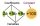Find the simplest form of the following expression: 3 to the 2nd power - 1/4 to the 2nd power.
• Five element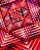The geometric sequence is given by quotient q = 1/2 and the sum of the first six members S6 = 63. Find the fifth element a5.
• Volume of woodEvery year, at the same time, an increase in the volume of wood in the forest is measured. The increase is regularly p% compared to the previous year. If in 10 years the volume of wood has increased by 10%, what is the number p?
• Mortage hypo loan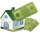The Jonáš family decided to buy an older apartment, which cost EUR 30,000. They found EUR 17,000 and took the loan with the bank for the remaining amount. What interest did they receive if they repay this amount for 15 years at EUR 120 per month?
• Cube constructionA 2×2×2 cube is to be constructed using 4 white and 4 black unit cube. How many different cubes can be constructed in this way? ( Two cubes are not different if one can be obtained by rotating the other. )
• A rectangle 2A rectangle has a diagonal length of 74cm. Its side lengths are in ratio 5:3. Find its side lengths.
• Before yesterdayHe merchant adds a sale sign in his shop window to the showed pair of shoes in the morning: "Today by p% cheaper than yesterday. " After a while, however, he decided that the sign saying: "Today 62.5% cheaper than the day before yesterday". Determine the
• Compound interest 3After 8 years, what is the total amount of a compound interest investment of \$25,000 at 3% interest, compounded quarterly? (interest is now dream - in the year 2019)

Do you have an exciting math question or word problem that you can't solve? Ask a question or post a math problem, and we can try to solve it.

We will send a solution to your e-mail address. Solved examples are also published here. Please enter the e-mail correctly and check whether you don't have a full mailbox.

Please do not submit problems from current active competitions such as Mathematical Olympiad, correspondence seminars etc...